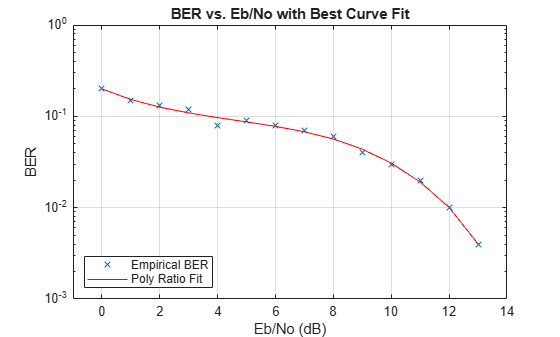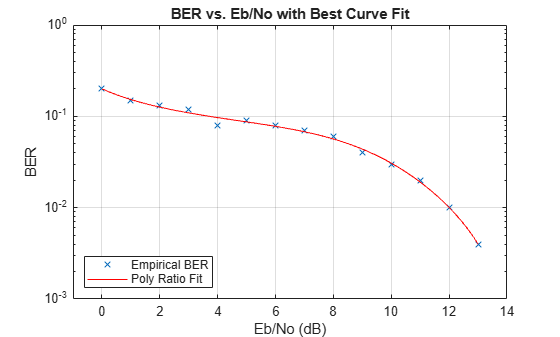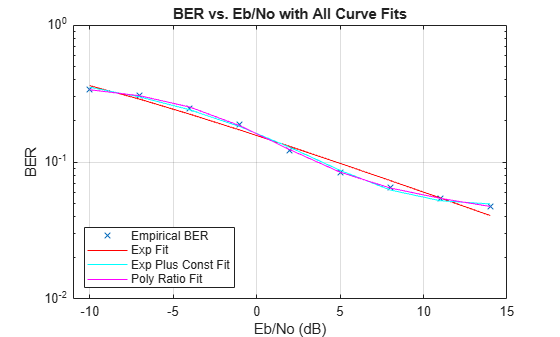# berfit

Fit curve to nonsmooth empirical BER data

## Syntax

``fitber = berfit(empEbNo,empber)``
``fitber = berfit(empEbNo,empber,fitEbNo)``
``fitber = berfit(empEbNo,empber,fitEbNo,options)``
``fitber = berfit(empEbNo,empber,fitEbNo,options,fittype)``
``[fitber,fitprops] = berfit(___)``
``berfit(___)``
``berfit(empEbNo,empber,fitEbNo,options,'all')``

## Description

example

````fitber = berfit(empEbNo,empber)` fits a curve to the empirical BER data, `empber`, and returns a vector of fitted BER points. The values in `empber` and `fitber` correspond to the empirical energy per bit to noise power spectral density ratio (Eb/N0) values given by `empEbNo`. For a general description of unconstrained nonlinear optimization, see . NoteThe `berfit` function is intended for curve fitting or interpolation (not extrapolation). Extrapolating BER data beyond an order of magnitude below the smallest empirical BER value is inherently unreliable. ```
````fitber = berfit(empEbNo,empber,fitEbNo)` specifies a vector of Eb/N0 values to use when fitting a curve to the empirical BER data in `empber` that correspond to the empirical Eb/N0 values in `empEbNo`.```
````fitber = berfit(empEbNo,empber,fitEbNo,options)` specifies a structure to override the default options used for optimization.```
````fitber = berfit(empEbNo,empber,fitEbNo,options,fittype)` specifies the closed-form function used to fit the empirical data. If you do not want to override the default options for optimization, specify `options` as [ ].```
````[fitber,fitprops] = berfit(___)` returns the `fitprops` structure with fields that describe the properties of the curve fit. Use any input argument combination from previous syntaxes.```
````berfit(___)` plots the empirical and fitted BER data.```
````berfit(empEbNo,empber,fitEbNo,options,'all')` plots the empirical and fitted BER data from all possible settings of `fittype` that are valid. If you do not want to override the default options for optimization, specify `options` as [ ]. NoteTo be valid a fit must conform to these criteria, otherwise it is rejected. real-valuedmonotonically decreasinggreater than or equal to 0 and less than or equal to 1 ```

## Examples

collapse all

This example shows the use of the `berfit` function using hard-coded or theoretical BER points for simplicity. For an example that uses empirical BER data from a simulation, see Use Curve Fitting on Error Rate Plot.

Best Fit for Set of Sample Data

Define a range of ${\mathit{E}}_{\mathrm{b}}/{\mathit{N}}_{0}$ values and BER points. Use this data as inputs for the `berfit` function.

```EbN0 = 0:13; berdata = [.2 .15 .13 .12 .08 .09 .08 .07 .06 .04 .03 .02 .01 .004]; berfit(EbN0,berdata); ```Plot Best Fit

The curve connects the points created by evaluating the fit expression at the specified ${\mathit{E}}_{\mathrm{b}}/{\mathit{N}}_{0}$ values. To make the curve look smoother, provide an input vector of ${\mathit{E}}_{\mathrm{b}}/{\mathit{N}}_{0}$ values for curve fitting in ascending order. This vector provides more points for plotting the curve and does not change the fit expression.

```fitEbNo = 0:0.2:13; berfit(EbN0,berdata,fitEbNo)```Fit for BER Curve with Error Floor

Run the `berfit` function using the `'all'` option on empirical BER results for a simulation of BPSK data transmitted over a channel with a null (`ch = [0.5 0.47]`) and recovered by using a linear MMSE equalizer at the receiver for the ${\mathit{E}}_{\mathrm{b}}/{\mathit{N}}_{0}$ range [-10, 15]. Comparing the results of curve fitting methods

• `'doubleExp+const'` fit type does not provide a valid fit

• `'exp'` fit type does not work well for this data

• `'exp+const'` and `'polyRatio'` fit types closely match the simulated data

```EbN0 = -10:3:15; empBER = [0.3361 0.3076 0.2470 0.1878 0.1212 0.0845 0.0650 0.0540 0.0474]; figure; berfit(EbN0,empBER,[],[],'all');```Use of `options` Input Structure and `fitprops` Output Structure

The `'notify'` value for the display level causes the function to produce output when one of the attempted fits does not converge. The `exitState` field of the output structure indicates which fit type converges.

Generate theoretical BER results for 8-PSK data with a diversity order of 2 transmitted over a Rayleigh fading channel for the ${\mathit{E}}_{\mathrm{b}}/{\mathit{N}}_{0}$ range [3, 10] dB.

```M = 8; EbN0 = 3:10; berdata = berfading(EbN0,'psk',M,2); % Compute the theoretical BER noisydata = berdata.*[.93 .92 1 .59 .08 .15 .01 .01];```

Create the `options` structure by using the `optimset` function to configure display and notification of fit type results. Run exponential and polynomial ratio fit types.

```options = optimset('display','notify'); disp('*** Trying exponential fit.') % Poor fit```
```*** Trying exponential fit. ```
```[fitber1,fitprops1] = berfit(EbN0,noisydata,EbN0,... options,'exp')```
``` Exiting: Maximum number of function evaluations has been exceeded - increase MaxFunEvals option. Current function value: 2.749919 ```
```fitber1 = 1×8 0.1247 0.0727 0.0376 0.0168 0.0064 0.0020 0.0005 0.0001 ```
```fitprops1 = struct with fields: fitType: 'exp' coeffs: [4x1 double] sumSqErr: 2.7499 exitState: 'The maximum number of function evaluations has been exceeded' funcCount: 10001 iterations: 6193 ```
`disp('*** Trying polynomial ratio fit.') % Good fit`
```*** Trying polynomial ratio fit. ```
```[fitber2,fitprops2] = berfit(EbN0,noisydata,EbN0,... options,'polyRatio')```
```fitber2 = 1×8 0.1701 0.0874 0.0407 0.0169 0.0060 0.0016 0.0003 0.0001 ```
```fitprops2 = struct with fields: fitType: 'polyRatio' coeffs: [6x1 double] sumSqErr: 2.3880 exitState: 'The curve fit converged to a solution' funcCount: 554 iterations: 331 ```

## Input Arguments

collapse all

Empirical Eb/N0 values in dB, specified as a vector with at least four elements. The element values in the vector must be in ascending order.

Data Types: `single` | `double`

Empirical BER data, specified as a vector with the same number of elements as input `empEbNo`. The values in `empber` correspond to the Eb/N0 values given by `empEbNo`.

Data Types: `single` | `double`

Eb/N0 values in dB for curve fitting, specified as a vector with element values in ascending order. The length of `fitEbNo` must equal or exceed that of input `empEbNo`.

Data Types: `single` | `double`

Override default options used for optimization, specified as a structure. The fields specified in the `options` structure are used by the `fminsearch` function. You can create the `options` structure by using the `optimset` function. This table describes the fields that are most relevant when using the `berfit` function. To use default options, you can specify this input as [ ].

FieldDescription
`options.Display`

Level of display.

• `'off'` (default) –- displays no output

• `'iter'` –- displays output at each iteration

• `'final'` –- displays only the final output

• `'notify'` –- displays output only if the function does not converge

`options.MaxFunEvals`The maximum number of function evaluations before optimization ceases. The default is 104.
`options.MaxIter`The maximum number of iterations before optimization ceases. The default is 104.
`options.TolFun`The termination tolerance for the closed-form function used to generate the fit. The default is 10-4.
`options.TolX`The termination tolerance for the coefficient values of the closed-form function used to generate the fit. The default is 10-4.

Data Types: `struct`

Closed-form function used to fit the empirical data, specified as `'exp'`, `'exp+const'`, `'polyRatio'`, or `'doubleExp+const'`. For more information, see Algorithms.

Data Types: `char` | `string`

## Output Arguments

collapse all

Fitted BER points, returned as a vector. The BER is computed for each Eb/N0 setting specified by the input `empEbNo` vector.

Data Types: `double`

Fit properties, returned as a structure with these fields to describe the properties of the curve fit.

FieldDescription
`fitprops.fitType`The closed-form function type used to generate the fit. Valid values include: `'exp'`, `'exp+const'`, `'polyRatio'`, or `'doubleExp+const'`.
`fitprops.coeffs`The coefficients used to generate the fit. If the function cannot find a valid fit, `fitprops.coeffs` is an empty vector.
`fitprops.sumSqErr`The sum squared error between the log of the fitted BER points and the log of the empirical BER points.
`fitprops.exitState`

The exit condition of `berfit`. Valid values include:

• `'The curve fit converged to a solution.'`

• ```'The maximum number of function evaluations was exceeded.'```

• `'No desirable fit was found.'`

`fitprops.funcCount`The number of function evaluations used in minimizing the sum squared error function.
`fitprops.iterations`The number of iterations taken in minimizing the sum squared error function. This value is not necessarily equal to the number of function evaluations.

Data Types: `struct`

## Algorithms

The `berfit` function fits the BER data using unconstrained nonlinear optimization via the `fminsearch` function. This table lists the closed-form functions that `berfit` considers based on the value of the `fittype` input argument. These functions were empirically found to provide close fits in a wide variety of situations, including exponentially decaying BERs, linearly varying BERs, and BER curves with error rate floors. In the functional expressions, x is a linear Eb/N0 value (not a dB value), and f(x) is the estimated BER.

`fittype` ValueFunctional Expression
`'exp'`

`$f\left(x\right)={a}_{1}\mathrm{exp}\left[\frac{-{\left(x-{a}_{2}\right)}^{{a}_{3}}}{{a}_{4}}\right]$`

`'exp+const'`

`$f\left(x\right)={a}_{1}\mathrm{exp}\left[\frac{-{\left(x-{a}_{2}\right)}^{{a}_{3}}}{{a}_{4}}\right]+{a}_{5}$`

`'polyRatio'`

`$f\left(x\right)=\frac{{a}_{1}{x}^{2}+{a}_{2}x+{a}_{3}}{{x}^{3}+{a}_{4}{x}^{2}+{a}_{5}x+{a}_{6}}$`

`'doubleExp+const'`

`$\begin{array}{l}{a}_{1}\mathrm{exp}\left[\frac{-{\left(x-{a}_{2}\right)}^{{a}_{3}}}{{a}_{4}}\right]\\ \begin{array}{cc}& \end{array}+{a}_{5}\mathrm{exp}\left[\frac{-{\left(x-{a}_{6}\right)}^{{a}_{7}}}{{a}_{8}}\right]+{a}_{9}\end{array}$`

The sum squared error function that `fminsearch` attempts to minimize is

The fitted BER points are the values in the output `fitber`, and the sum is over the Eb/N0 points given in the input `empEbNo`. To avoid high-BER regions dominating the objective function, the sum squared equation uses the log of the BER values rather than the BER values themselves.

 Chapra, Steven C., and Raymond P. Canale. Numerical Methods for Engineers. Fourth Edition. New York, McGraw-Hill, 2002.